# Electronics and Communication Engineering - Exam Questions Papers

16.

A conductor in x-y plane and having length 1 m is moving with a velocity V = (2i + 3j + k) m/sec. A magnetic induction field B = (i + 2j) Wb/m2 is applied to the conductor. The potential difference between the ends of the conductor is

 A. 0 B. 4.88 V C. 6 V D. None of these

Explanation: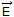= - (V x B) = - (2i + 3j + k) x (i + 2j)

|| = (E2x + E2y + E2z) = 6 v/m

Potential Difference across 1 m length = 6.

17.

A 1000 kHz carrier wave modulated 40% at 4000 Hz is applied to a resonant circuit tuned to a carrier frequency and having Q = 140. What is the degree of modulation after passing the wave through this circuit?

 A. 0.4 B. 0.2 C. 0.27 D. 0.554

Explanation:

Resulting depth of modulation is given by :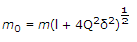when δ =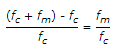fc = 1000 x 103 Hz

fm = 4 x 103 Hz

δ =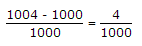m0 =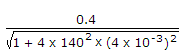=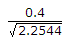= 0.27 .

18.

The VTH at terminals A and B is equal to,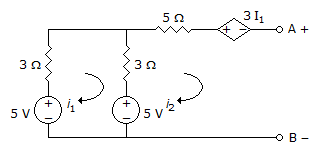A. 0 V B. 5 V C. - 5 V D. None of these

Explanation:

Apply KVL to loop 1

5V - 3I1 - 2(I1 - I2) - 5V = 0

I2 = 0

5 - 3I1 - 2I1 - 5V = 0

I1 = 0

VAB = 5V.

19.

If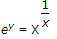, then y has a

 A. maximum at x = e B. minimum at x = e C. maximum at x = e-1 D. minimum at x = e-l

Explanation: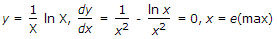.

20.

If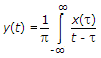. dt, then F[y(t)] is

 A. - jsgn(f). x(f) B. j2pf . e-jptf . x(f) C.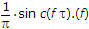D.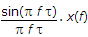Explanation: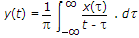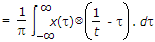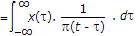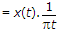⇒ F{Y(t)} = Y(f) = X(f) x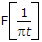sin (t) ⇌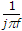By duality :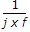⇌ sgn + (- f)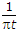jsgn (- f)⇌ - j sgn (f)

∵ sgn(- f) = - sgn(f)

⇒ Y(f) = X(f). [- j sgn(f)].

#### Current Affairs 2022

Interview Questions and Answers